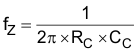SLVSDA7E February   2017  – August 2019

PRODUCTION DATA.

1. Features
2. Applications
3. Description
1.     Device Images
4. Revision History
5. Device Comparison Table
6. Pin Configuration and Functions
7. Specifications
8. Detailed Description
1. 8.1 Overview
2. 8.2 Functional Block Diagram
3. 8.3 Feature Description
4. 8.4 Device Functional Modes
9. Application and Implementation
1. 9.1 Application Information
2. 9.2 Typical Application
1. 9.2.1 Design Requirements
2. 9.2.2 Detailed Design Procedure
3. 9.2.3 Setting the Current Limit
4. 9.2.4 Setting the Output Voltage
5. 9.2.5 TPS61178 Application Waveform
3. 9.3 System Examples
10. 10Power Supply Recommendations
11. 11Layout
12. 12Device and Documentation Support
1. 12.1 Device Support
2. 12.2 Documentation Support
5. 12.5 Community Resources
7. 12.7 Electrostatic Discharge Caution
8. 12.8 Glossary
13. 13Mechanical, Packaging, and Orderable Information

• RNW|13

#### 9.2.4.4.1 Small Signal Model

The TPS61178x uses the fixed frequency peak current mode control; there is an internal adaptive slope compensation to avoid the sub-harmonic oscillation. With the inductor current information sensed, the small-signal model of the power stage reduces from a two-pole system, created by L and COUT, to a single-pole system, created by ROUT and COUT. The single-pole system is easily used with the loop compensation. Figure 24 shows the equivalent small signal elements of a boost converter.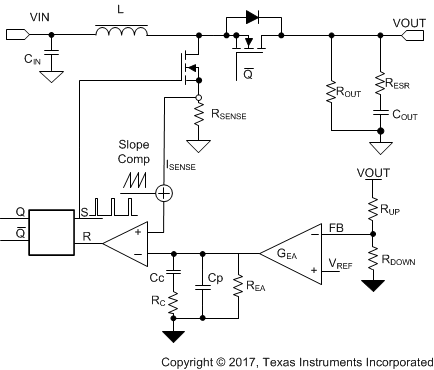Figure 24. TPS61178x Control Equivalent Circuitry Model

The small signal of power stage including the slope compensation is:

Equation 15.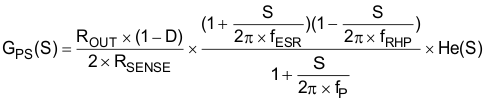where

• D is the duty cycle
• ROUT is the output load resistor
• RSENSE is the equivalent internal current sense resistor, which is typically 0.083 Ω of TPS61178x

The single pole of the power stage is:

Equation 16.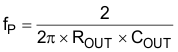where

• COUT is the output capacitance, for a boost converter having multiple, identical output capacitors in parallel, simply combine the capacitors with the equivalent capacitance

The zero created by the ESR of the output capacitor is:

Equation 17.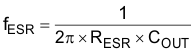where

• RESR is the equivalent resistance in series of the output capacitor.

The right-hand plane zero is:

Equation 18.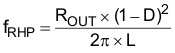where

• D is the duty cycle
• ROUT is the output load resistor
• L is the inductance

Using He(s) to model the inductor current sampling effect as well as the slope compensation effect on the small signal response, is shown in Equation 19

Equation 19.Equation 20.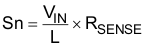where

• Sn is the slew rate of the inductor current ramping up

Equation 21.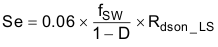where

• Se is the slope compensation slew rate
• Rdson_LS is the on resistance of Low-side FET

The slope compensation adaptively changes with the switching frequency and duty cycle.

He(s) models the inductor current sampling effect as well as the slope compensation effect on the small signal response. Note that if Sn > Se, e.g., when L is too small, the converter operates as a voltage mode converter and the above model no longer holds.

The TPS61178x COMP pin is the output of the internal trans-conductance amplifier.

Equation 22 shows the equation for feedback resistor network and the error amplifier.

Equation 22.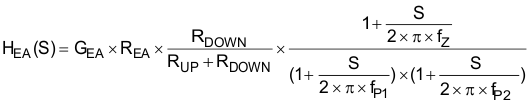where

• kCOMP and REA are the ratio of peak current / comp voltage, for TPS61178x, the typical value is kCOMP = 12 A / V and REA = 20 MΩ.
• ƒP1, ƒP2 is the pole's frequency of the compensation, fZ is the zero’s frequency of the compensation network. network
Equation 23.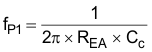where

• CC is the zero capacitor compensation
Equation 24.where

• CP is the pole capacitor compensation
• RC is the resistor of the compensation network
Equation 25.